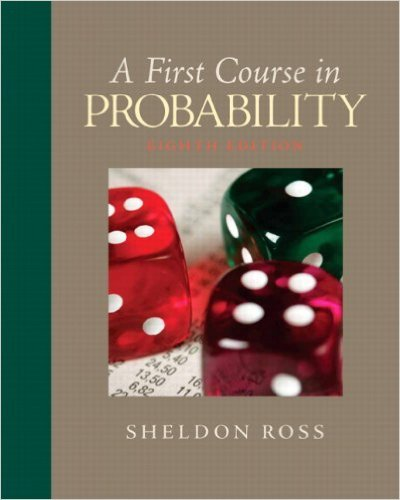×
Get Full Access to First Course In Probability - 8 Edition - Chapter 2 - Problem 3
Get Full Access to First Course In Probability - 8 Edition - Chapter 2 - Problem 3

×

# Two dice are thrown. Let E be the event that thesum of theISBN: 9780136033134 163

## Solution for problem 3 Chapter 2

First Course in Probability | 8th Edition

• Textbook Solutions
• 2901 Step-by-step solutions solved by professors and subject experts
• Get 24/7 help from StudySoup virtual teaching assistantsFirst Course in Probability | 8th Edition

4 5 1 250 Reviews
11
4
Problem 3

Two dice are thrown. Let E be the event that thesum of the dice is odd, let F be the event thatat least one of the dice lands on 1, and let G bethe event that the sum is 5. Describe the eventsEF,E F,FG,EFc, and EFG.

Step-by-Step Solution:
Step 1 of 3

X, Y Bivariate Random Variables f(u1, 2 ) is density of (X,Y) ∫ ( ) density of X ∫ ( ) density of Y X, Y are independent if f(u1, 2 = f1(u1 2 (u2) If X1, ,2…….X are nndependent Var(X 1 ……..X ) = n + …….. We want to produce to specification “m” “m” = length of a part, content of juice can etc We want to check at the end of the assembly line, if we are meeting the quality of the product (Quality-Control) Process: We choose a sample of size “n” from the product X 1 X 2 …….X n are independent random variables. They have the same distribution. Look at the average of the If X is a random variable then Var(aX) Var( ) = = = ( We

Step 2 of 3

Step 3 of 3

##### ISBN: 9780136033134

Unlock Textbook Solution